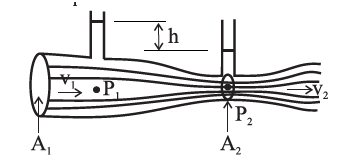+1 vote
88 views

Why does the speed of a liquid increase and its pressure decrease when a liquid passes through constriction in a horizontal pipe?

| 88 views

Consider a horizontal constricted tube.Let Aand A2 be the cross-sectional areas at points P1 and P2, respectively.

Let v1 and v2 be the corresponding flow speeds.

ρis the density of the fluid in the pipeline.

By the equation of continuity,

A1v= A2v2     ... (1)

v2/ v1 =A1/A> 1    (…A1>A2)

Therefore, the speed of the liquid increases as it passes through the constriction. Since the meter is assumed to be horizontal, from Bernoulli’s equation we get,

$$p_1+\frac{1}{2}ρv_1^2$$ =$$p_2+\frac{1}{2}ρv_2^2$$

$$p_1+\frac{1}{2}ρv_1^2$$ =$$p_2+\frac{1}{2}ρv_1^2(\frac{A_1}{A_2})^2$$

$$p_1-p_2=\frac{1}{2}ρv_1^2\left[(\frac{A_1}{A_2})^2-1\right]$$

Again, since A1>Athe bracketed term is positive so that p1 > p2. Thus, as the fluid passes through the constriction or throat, the higher speed results in lower pressure at the throat.

by Expert (11.6k points)